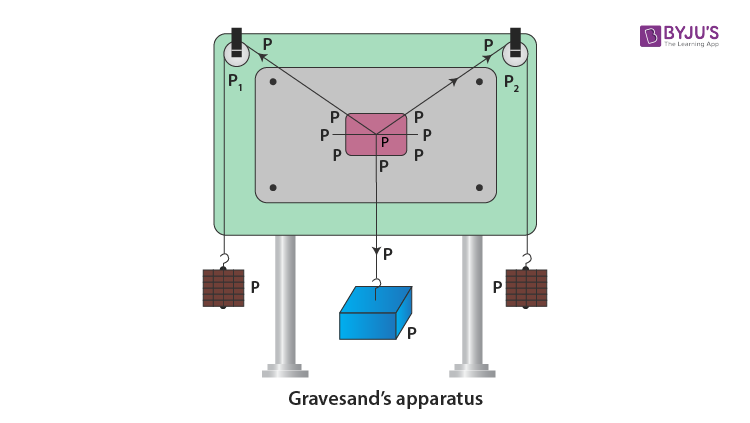Checkout JEE MAINS 2022 Question Paper Analysis : Checkout JEE MAINS 2022 Question Paper Analysis :

# To Find The Weight Of A Given Body Using Parallelogram Law Of Vectors

## Aim

To find the weight of a given body using the parallelogram law of vectors.

## Materials Required

1. Gravesand’s apparatus which is a parallelogram law of forces apparatus
2. Plumb line
3. Two hangers with slotted weights
4. A body whose weight is to be determined
6. White drawing paper
7. Drawing pins
8. Mirror strip
9. Sharp pencil
10. Half-meter scale
11. Set squares
12. Protractor

## Theory

If an unknown weight body S is suspended from the middle of the hanger, and P and Q are the two balance weights from the other two ends of the hanger, then the unknown weight is calculated using the below equation:

 $$\begin{array}{l}S=\sqrt{P^{2}+Q^{2}+2PQcos\theta }\end{array}$$

Where,

• S is the unknown weight
• P and Q are the balance weights

## Diagram## Procedure

1. Set up the Gravesand’s apparatus with its board vertical and with the help of a plumb line.
2. Oil the pulleys P1 and P2 if they are not friction-less.
3. With the help of drawing pins, fix the white sheet on the board.
4. Let O be the knot made from three-piece of strong thread.
5. Let P and Q be the weights that are tied at both the ends of the hanger and S be the given body tied to the third end.
6. Let the third weight S be vertically in the middle of the board and weights P and Q are passed over the pulley.
7. Junction O should be maintained at equilibrium by adjusting weights P and Q.
8. All the three weights P, Q and S act as three forces
$$\begin{array}{l}\underset{P}{\rightarrow}\end{array}$$
,
$$\begin{array}{l}\underset{Q}{\rightarrow}\end{array}$$
and
$$\begin{array}{l}\underset{S}{\rightarrow}\end{array}$$
.
9. All three weights should hang freely without touching the board or table.
10. With a sharp pencil, mark the position of junction O.
11. Disturb the weights P and Q and leave them free.
12. The position of junction O will be closer to the earlier position.
13. Let P1 and P2 be the position of P, Q1 and Q2 be the position of Q and S1 and S2 be the position of S which are taken down with the help of the mirror.
14. Remove the paper from the board.
15. Using half-meter scale draw lines through P1 and P2, Q1 and Q2 and S1 and S2 represent P, Q, and S respectively. All these lines should meet at point O.
16. Taking a scale, 1 cm = 50 g, OA = 3 cm and OB = 3 cm to represent P =150g and Q = 150g.
17. R is represented by completing the parallelogram OACB and by joining OC with the help of set squares.
18. When OC is measured, it comes to 3.9 cm.
19. P and Q can be changed for different sets.
20. By using spring balance, find the weight of the wooden box.

## Observation

Least count of spring balance = …… g

Zero error of spring balance = …….. g

Weight of unknown body by spring balance = …….g

Scale used: Let 1 cm = 50 g

 Sl. no Forces Sides Resultant force R (g wt) Unknown weight S (g wt) Weight by spring balance (g wt) Error (g wt) P (g wt) Q (g wt) OA (cm) OB (cm) OC (cm) 1. 150 150 3 3 3.9 195 195 200 5 2. 3.

## Calculations

OC = 3.9 cm, R = 50 × 3.9 = 195g

Unknown weight, S = 195g

Mean unknown weight,

$$\begin{array}{l}S=\frac{S_{1}+S_{2}+S_{3}}{3}\end{array}$$
= 195g

Weight by spring balance = 200g

Difference = 5g

## Result

The unknown weight of given body = 195g

The error is within limits of experimental error.

## Precautions

1. The board should be stable and placed verticle.
2. There shouldn’t be any friction in the pulleys.
3. The board or the table should not be in touch with the hangers.
4. The middle of the paper should have junction O.
5. When the weights are at rest, the points should be marked.
6. A sharp pencil should be used to mark the points.
7. To show the direction of forces, arrows should be marked.
8. To make a fairly big parallelogram, a proper scale should be taken.

## Sources Of Error

1. There might be friction in the pulleys.
2. Weights might not be accurate.
3. Points marked may be correct.
4. Weight measured by spring balance may not be accurate.

## Viva Questions

Q1. Define scalar quantity.

Ans: It is defined as the physical quantity which has only magnitude like length, mass, etc.

Q2. Define vector quantity.

Ans: It is defined as the physical quantity which has magnitude and direction like force, velocity, etc.

Ans: The addition of vector is defined as the operation of adding two or more vectors together into a vector sum.

Q4. What is resolution of vectors?

Ans: Resolution of the vector is defined as the breaking of a single vector into its components.

Q5. Why is addition of vector different from the addition of scalars?

Ans: Addition of vector has direction and magnitude whereas the addition of scalar has only magnitude. Therefore, the addition of vector is different from the addition of scalar.